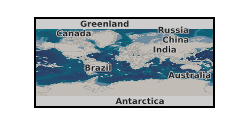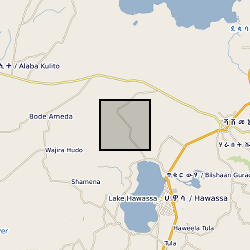Keyword

## Magma

12 record(s)

Type of resources
Topics
Keywords
Contact for the resource
Provided by
Years
Formats
Representation types
Update frequencies
From 1 - 10 / 12
• # Rheology of three-phase suspensions (NERC grant NE/K500999/1)The data consists of a spreadsheet containing rheology data for 39 samples of syrup, containing air bubbles and/or spherical glass particles. These data were used by Truby et al. (2014) to support a model for the rheology of a three-phase suspension. Each sample was placed in the rheometer (concentric cylinder geometry), and the stress was stepped up and then down, taking a measurement of strain rate at each step. Further details of the experiments may be found in Truby et al. (2014). NERC grant is NE/K500999/1. Co-author working with a NERC grant, NE/G014426/1.

• # Bubble growth in silicate melts (NERC Grant NE/N002954/1)Vesicularity (phi) as a function of time for samples of natural hydrated silicate glass (obsidian) from optical dilatrometric analysis. Also numerical model for analysis of dataset and associated user guide.

• # XRheo development data (NERC grant NE/M018687/1)Reconstructed data - This dataset contains the reconstructed image data. Each sub-folder contains a set of 2D slices that together make up a 3D image from that time point. Not all images from all datasets have been reconstructed, the values in parentheses refer to the scan numbers that have been reconstructed. Raw data - This dataset contains the raw unprocessed image data collected during the development of the XRheo system. Processed data - This dataset contains the post-processing outputs from analysis of the data from the XRheo development experiments. Each sub-folder contains the files generated during filtering, segmentation and separation of the features [M (melt), B (bubbles), X (crystals)], and the post processing analysis for size distributions and tracking. The data sets included are the results of dynamic X-ray tomography experiments performed on multiphase synthesised magmas being deformed under known temperature and strain rates for a concentric cylinder geometry.

• # Numerical calculations of compacting flow past spheres and circular cylinders (NERC grant NE/I023929/1)This data contains the results of numerical simulations described in the following two papers: Alisic L., Rhebergen S., Rudge J.F., Katz R.F., Wells G.N. Torsion of a cylinder of partially molten rock with a spherical inclusion: theory and simulation (2016) Geochem. Geophys. Geosyst.16 doi:10.1002/2015GC006061 Alisic L., Rudge J.F., Katz R.F., Wells G.N., Rhebergen S. Compaction around a rigid, circular inclusion in partially molten rock (2014) J. Geophys. Res. Solid Earth 119:5903-5920 doi:10.1002/2013JB010906

• # Three-Field block preconditioner 3D test case (NERC grant NE/I026995/1)This data is described in section 6.4 of the following paper, Three-Field Block Preconditioners for Models of Coupled Magma/Mantle Dynamics by Rhebergen et al DOI:10.1137/14099718X

• # Evidence for the top-down control of lava domes on magma ascent dynamics. (NERC Grant ref NE/L002574/1)Data output from the numerical flow modelling in GRL manuscript ""Evidence for the top-down control of lava domes on magma ascent dynamics"", by Marsden, L., Neuberg, J. & Thomas, M., all of University of Leeds. The models were created using the Laminar Flow module in COMSOL Multiphysics v5.4 by L. Marsden. The following files are uploaded: Archive_Reference_Model.txt (Reference flow model: Gas loss function, Initial H2O content = 4.5 wt.% Excess pressure at depth = 10 MPa, Constant corresponding to crystal growth rate = 4e-6 s^-1 ) Archive_High_H2O.txt (Gas loss function, Initial H2O content = 10 wt.% Excess pressure at depth = 10 MPa, Constant corresponding to crystal growth rate = 4e-6 s^-1) Archive_No_Gas_Loss.txt (No gas loss, Initial H2O content = 4.5 wt.% Excess pressure at depth = 10 MPa, Constant corresponding to crystal growth rate = 4e-6 s^-1) Archive_Gamma_Low.txt (Gas loss function, Initial H2O content = 4.5 wt.% Excess pressure at depth = 10 MPa, Constant corresponding to crystal growth rate = 1e-6 s^-1) Archive_Excess_Pressure_0MPa.txt (Gas loss function, Initial H2O content = 4.5 wt.% Excess pressure at depth = 0 MPa, Constant corresponding to crystal growth rate = 4e-6 s^-1) Archive_Excess_Pressure_20MPa.txt (Gas loss function, Initial H2O content = 4.5 wt.% Excess pressure at depth = 20 MPa, Constant corresponding to crystal growth rate = 4e-6 s^-1) The files uploaded include the reference flow model and where a single key parameter has been changed in the flow modelling. We include data where the key parameter is at the upper or lower limit of the values tested. Data are not included where magma ascent is modelled to stall without the extrusion of a lava dome, as a time dependent model is not run in this case. A solution is provided using equilibrium modelling only. The following variables are output, at conduit centre unless specified: Depth (m), Time(s), Ascent velocity (m/s), Bulk Viscosity (Pa s), Crystal Content, Dome height (m), Gas Volume Fraction, Overpressure (Pa), Shear Stress at Conduit Wall (Pa)

• # Experimental petrology and monitoring observations from the 2014 Kelud, Indonesia (NERC grant NE/N014286/1)Here, we provide data corresponding to the experimental conditions used, the results gained via electron microprobe for natural and experimental volcanic samples. Mass balance calculations and a compilation of monitoring data for recent explosive eruptions.

• # Compaction in the Skaergaard Intrusion (NERC grant NE/J021520/1)Electron backscatter diffraction data for cumulates from the Skaergaard Intrusion of East Greenland. 12 samples from the Skaergaard Intrusion: 9 from the Layered Series, and 3 from the trough layering. Layered Series samples have a prefix LS; Trough layer samples have a prefix TB.

• # Time-lapse gravity data Corbetti caldera (Ethiopia): 2014/16 (NERC grant NE/L013932/1)The RiftVolc microgravity network was comprised of a total of 4 benchmarks including a reference benchmark. Benchmark locations, observed gravity changes, dg14 -16, from 2014-2016, corresponding vertical deformation, Uz, free-air effect, and resultant residual gravity changes gr of the microgravity and GNSS network at Corbetti.

• # Supporting data for 'DFENS: Diffusion chronometry using Finite Elements and Nested Sampling' (NERC Grant NE/L002507/1)This is supporting data for the manuscript entitled 'DFENS: Diffusion chronometry using Finite Elements and Nested Sampling' by E. J. F. Mutch, J. Maclennan, O. Shorttle, J. F. Rudge and D. Neave. Preprint here: https://doi.org/10.1002/essoar.10503709.1 Data Set S1. ds01.csv Electron probe microanalysis (EPMA) profile data of olivine crystals used in this study. Standard deviations are averaged values of standard deviations from counting statistics and repeat measurements of secondary standards. Data Set S2. ds02.csv Plagioclase compositional profiles used in this study, including SIMS, EPMA and step scan data. Standard deviations for EPMA analyses are averaged values of standard deviations from counting statistics and repeat measurements of secondary standards. Standard deviations for SIMS and step scan analyses are based on analytical precision of secondary standards. Data Set S3. ds03.csv Angles between the EPMA profile and the main olivine crystallographic axes measured by electron backscatter diffraction (EBSD). 'angle100X' is the angle between the  crystallographic axis and the x direction of the EBSD map, 'angle100Y' is the angle between  crystallographic axis and the y direction of the EBSD map, and 'angle100Z' is the angle between the  crystallographic axis and the z direction in the EBSD map etc. 'angle100P' is the angle between the EPMA profile and the  crystallographic axis, 'angle010P' is the angle between the EPMA profile and the  crystallographic axis, and 'angle100P' is the angle between the EPMA profile and the  crystallographic axis. All angles are in degrees. Data Set S4. ds04.csv Median timescales and 1 sigma errors from the olivine crystals of this study. The +1 sigma (days) is the quantile value calculated at 0.841 (i.e. 0.5 + (0.6826 / 2)). The -1 sigma (days) is therefore the quantile calculated at approximately 0.158 (which is 1 - 0.841). The 2 sigma is basically the same but it is 0.5 + (0.95/2). The value quoted as the +1 sigma (error) is the difference between the upper 1 sigma quantile and the median. Likewise the -1 sigma (error) is the difference between the median and the lower 1 sigma quantile. Data Set S5. ds05.xlsx Median timescales and 1 sigma errors from the plagioclase crystals of this study. Results from each of the parameterisations of the Mg-in-plagioclase diffusion data are included: Faak et al, (2013), Van Orman et al., (2014) and a combined expression. Data Set S6. ds06.xlsx Spreadsheet containing the regression parameters and covariance matrices used in this study and in Mutch et al. (2019). Additional versions of the olivine regressions where the ln fO2 is expressed in Pa have been made for completeness. We recommend using the versions where ln fO2 is expressed in its native form (bars).